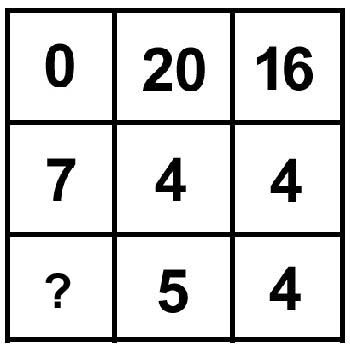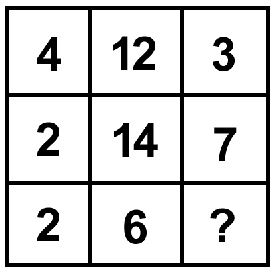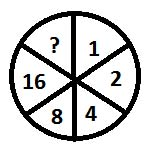0
6890

# RRB NTPC Number Series Questions PDF

Download RRB NTPC Number Series Questions and Answers PDF. Top 25 RRB NTPC Number Series questions based on asked questions in previous exam papers very important for the Railway NTPC exam.Question 1: Choose the incorrect number in the series: 31, 37, 41, 43, 47, 51, 53

a) 53

b) 51

c) 47

d) 43

Question 2: A series is given with one missing term. Choose the correct alternative from the given optons that will complete the series.
17, 42, 78, 127, 191, ?

a) 272

b) 268

c) 275

d) 271

Question 3: Find the number which should replace ‘?’.
320, 480, 720, 1080, ?

a) 1121

b) 1728

c) 1620

d) 1628Question 4: Choose the incorrect number in the series: 12, 13, 28, 87, 350, 1765

a) 28

b) 87

c) 350

d) 1765

Question 5: Choose the incorrect number in the given series: 1, 3, 6, 10, 15, 22

a) 22

b) 15

c) 10

d) 6

Question 6: Find the incorrect number in the following series: 12, 17, 25, 36, 50, 68

a) 25

b) 36

c) 50

d) 68

Question 7: Find the incorrect term in the sequence below –
84, 72, 61, 52, 42, 34

a) 61

b) 52

c) 34

d) 84

Question 8: Find the number which will replace ‘?’.
31, 71, 91, 32, 92, ?

a) 13

b) 39

c) 101

d) 73

Question 9: A series is given with one missing term. Choose the correct alternative from the given optons that will complete the series.
784, 685, 590, 499, 412, ?

a) 317

b) 345

c) 329

d) 351

Question 10: Find the number which would replace the question mark in the series given below.
13, 17, 25, 32, 37, ?

a) 47

b) 43

c) 45

d) 44

Question 11: Find the next number in the series.
22, 29, 43, 64, ?

a) 86

b) 85

c) 87

d) 92

Question 12: Find the missing number 23,26,32,41,53,68,??

a) 81

b) 84

c) 76

d) 86Question 13: 13,17,30,47,77,124,??

a) 191

b) 201

c) 189

d) 213

Question 14: What number comes in the place of the question mark?a) 0

b) -1

c) 1

d) 2

Question 15: a, b and c are in Geometric progression such that c – a = 12 and b + c = 24. What is the value of (b+1)(b-1)?

a) 80

b) 63

c) 48

d) 35

Question 16: What number comes in the place of the question mark?a) 0

b) -1

c) 1

d) 2

Question 17: What number comes in the place of the question mark?a) 1

b) 3

c) 4

d) 5

Question 18: Which of the following is a prime number?

a) 87

b) 91

c) 93

d) 97

Question 19: What will come in the following question in place of the question mark ?a) 32

b) 18

c) 22

d) 28

Question 20: Find the next term in the series: 81, 121, 169, 225, 289, ?

a) 441

b) 361

c) 391

d) 484Question 21: What is the sum to 30 terms of the series: 6, 12, 18, 24,… ?

a) 2800

b) 2780

c) 2690

d) 2790

Question 22: Find the next term in the series: 12, 18, 26, 36, 48, 62, ?

a) 80

b) 74

c) 76

d) 78

Question 23: Which number comes next in the following series: 5, 15, 35, 65, 105, 155, ?

a) 195

b) 215

c) 225

d) 235

Question 24: Find the wrong number in the given series
132, 90, 48, 30, 12, 2

a) 132

b) 48

c) 30

d) 2

Question 25: Find the next number in the series:
3, 15, 35, 63, 99, ___

a) 123

b) 137

c) 143

d) 159The series lists all the prime numbers from 31 in ascending order.
51 is not a prime number. It is divisible by 17. Therefore, 51 is the incorrect term in the series.

17 + 25 = 42
42 + 36 = 78
78 + 49 = 127
127 + 64 = 191
191 + 81 = 272
Hence, option A is the right answer.

The series follows the following pattern:
320
320 * 1.5 = 480
480 * 1.5 = 720
720 * 1.5 = 1080
1080* 1.5 = 1620

12*1 + 1 = 12+1 = 13
13*2 + 2 = 26+2 = 28
28*3 + 3 = 84 +3= 87
87*4+4 = 348+4 = 352
352*5+5 = 1760+5=1765

As we can see, 350 is the incorrect term in the series. Therefore, option C is the right answer.

The given numbers are of the form n(n+1)/2.

1*2/2 = 1*1 = 1

2*3/2 = 1*3 = 3

3*4/2 = 3*2 = 6

4*5/2 = 2*5 = 10

5*6/2 = 5*3 = 15

6*7/2 = 3*7 = 21

As we can see, 22 is the incorrect term. Therefore, option A is the right answer.

17 – 12 = 5
25 – 17 = 8
36 – 25 = 11
50 – 36 = 14
As we can see, the difference between 2 consecutive numbers in the series are in an AP.
The next term must be 50 + 17 = 67.
Therefore, 68 is the incorrect term in the series and hence, option D is the right answer.

84 – 12 = 72
72 – 11 = 61
61 – 10 = 51
51 – 9 = 42
42 – 8 = 34
Hence, option B is the right answer.

All the prime numbers from 13 are written in reverse order.
i.e 13, 17, 19, 23, 29, 31,..
are written as 31, 71, 91, 32, 92, 13

784 – 99 = 685
685 – 99 + 4 = 590
590 – 99 + 8 = 499
499 – 99 + 12 = 412
412 – 99 + 16 = 329
Hence, option C is the right answer.The pattern being followed here is that the sum of the digits of the previous number is being added to get the next number. For example:
13 + 1 + 3 = 17
17 + 1 + 7 = 25
25 + 2+ 5 = 32
32 + 3 + 2 = 37
Following the same pattern, the next term of the sequence will be
37 + 3 + 7 = 47
Hence, option A is correct.

The series follows the following pattern:
$22+7=29$
$29 + 14 = 43$
$43 + 21 = 65$
$64 + 28 = 92$

The numbers given above are part of a pattern given below.

26 = 23 + 3
32 = 26 + 6
41 = 32 + 9
53 = 41 + 12
68 = 53 + 15
Hence, the number to follow will be 68 + 18 = 86

The numbers given in the series above follow the below pattern

30 = 17 + 13
47 = 30 + 17
77 = 47 + 30
124 = 77 + 47
Therefore, the number to replace the question mark is 124 + 77 = 201

In the right column, 16 = 4 * 4
In the middle column, 20 = 4 * 5
In the left column, 0 = 7 * ?
So, ? = 0

Let the common ratio be r
So, b = ar
And c = $ar^2$
So, $ar^2$ – a = 12
a(r+1)(r-1) = 12 …. (1)
ar + $ar^2$ = 24
ar(1+r) = 24 ….. (2)

(2) / (1) => r/(r-1) = 2
r = 2r – 2
r = 2
From (2), a * 2 * (2+1) = 24.
So, a = 4
(b+1) (b-1) = (ar+1)(ar-1) = (4*2 + 1)(4*2-1) = 63

In the right column, 16 = 4 * 4
In the middle column, 20 = 4 * 5
In the left column, 0 = 7 * ?
So, ? = 0

In the first row, 4 * 3 = 12
In the second row, 2 * 7 =14
In the third row, 2 * ? = 6.
So, ? = 3

87 and 93 are divisible by 3. 91 is divisible by 7. Only 97 is a prime number.

the sequence is 1,1×2=2 , 4×2=8, 8×2 =16 so the next in sequence is 16×2=32

The terms in the series are $9^2$, $11^2$, $13^2$, $15^2$ and so on. So, the next term in the series is $19^2 = 361$

The terms of the series are in Arithmetic progression. So, the sum to n terms is given by S = (n/2) * [2a +(n-1)d]
So, the sum in this case is S = (30/2) * [2*6 + 29*6] = 15 * [12 + 174] = 15 * 186 = 2790

The difference between successive terms is 6, 8, 10, 12, 14 and so on.
So, the next term is 62 + 16 = 78

The difference between the first two numbers is 10. The difference between the second and third numbers is 20 and so on. So, the next number in the series will be 155 + 60 = 215Each term is $(2n)^2-1$. Hence, the 6th term = $(2*6)^2-1$ =144-1=143.